# How to show formulas in Excel

In this short tutorial, you will learn an easy way to display formulas in Excel 2016, 2013, 2010 and older versions. Also, you will learn how to print formulas and why sometimes Excel shows a formula, not result, in a cell.

If you are working on a spreadsheet with a lot of formulas in it, it may become challenging to comprehend how all those formulas relate to each other. Showing formulas in Excel instead of their results can help you track the data used in each calculation and quickly check your formulas for errors.

Microsoft Excel provides a really simple and quick way to show formulas in cells, and in a moment, you will make sure of this.

## How to show formulas in Excel

Usually, when you enter a formula in a cell and press the Enter key, Excel immediately displays the calculated result. To show all formulas in the cells containing them, use one of the following methods.

#### 1. Show Formulas option on the Excel ribbon

In your Excel worksheet, go to the Formulas tab > Formula Auditing group and click the Show Formulas button.

Microsoft Excel displays formulas in cells instead of their results right away. To get the calculated values back, click the Show Formulas button again to toggle it off.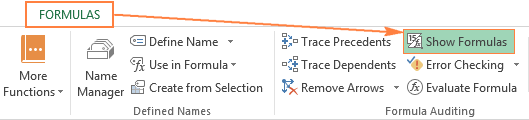#### 2. 'Show formulas in cells instead of their results' option

In Excel 2010, Excel 2013 and Excel 2016, go to File > Options. In Excel 2007, click Office Button > Excel Options.

Select Advanced on the left pane, scroll down to the Display options for this worksheet section and select the option Show formulas in cells instead of their calculated results.

At first sight, this seems to be a longer way, but you may find it useful when you want to display formulas in a number of Excel sheets, within the currently open workbooks. In this case, you just select the sheet name from the dropdown list and check the Show formulas in cells… option for each sheet.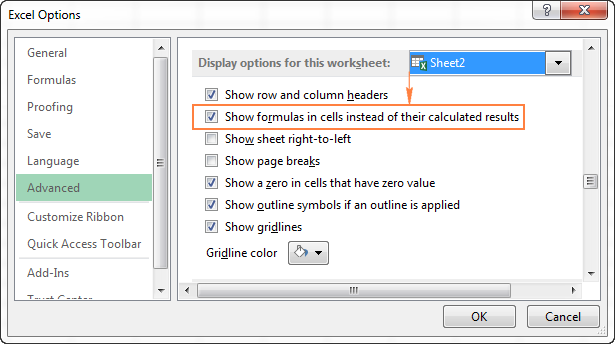#### 3. Excel shortcut to show formulas

The fastest way to see every formula in your Excel spreadsheet is pressing the following shortcut: Ctrl + `

The grave accent key (`) is the furthest key to the left on the row with the number keys (next to the number 1 key).

The Show Formulas shortcut toggles between displaying cell values and cell formulas. To get the formula results back, simply hit the shortcut again.

Note. Whichever of the above methods you use, Microsoft Excel will show all formulas of the current worksheet. To display formulas in other sheets and workbooks, you will need to repeat the process for each sheet individually.

If you want to view the data used in a formula's calculations, use any of the above methods to show formulas in cells, then select the cell containing the formula in question, and you will see a result similar to this: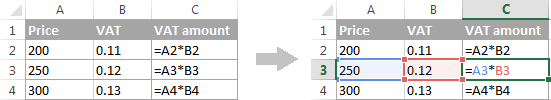Tip. If you click a cell with a formula, but the formula does not show up in the formula bar, then most likely that formula is hidden and the worksheet is protected. Here are the steps to unhide formulas and remove the worksheet protection.

If you want to print formulas in your Excel spreadsheet instead of printing the calculated results of those formulas, just use any of the 3 methods to show formulas in cells, and then print the worksheet as you normally print your Excel files (File > Print). That's it!

## Why is Excel showing formula, not result?

Did it ever happen to you that you type a formula in a cell, press the Enter key… and Excel still shows the formula instead of the result? Don't worry, your Excel is all right, and we will have that mishap fixed in a moment.

In general, Microsoft Excel can display formulas instead of calculated values for the following reasons:

1. You may have inadvertently activated the Show Formulas mode by clicking the corresponding button on the ribbon, or pressing the CTRL+` shortcut. To get the calculated results back, just toggle off the Show Formulas button or press CTRL+` again.
2. You may have accidentally typed a space or single quote (') before the equal sign in the formula: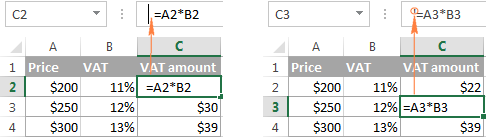When a space or single quote precedes the equal sign, Excel treats the cell contents as text and does not evaluate any formula within that cell. To fix this, just remove the leading space or single quote.

3. Before entering a formula in a cell, you may have set the cell's formatting to Text. In this case, Excel also perceives the formula as a usual text string and does not calculate it.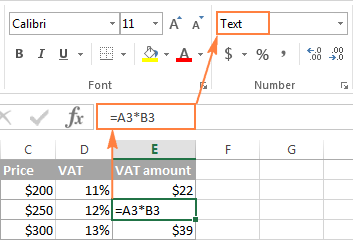To fix this error, select the cell, go to the Home tab > Number group, and set the cell's formatting to General, and while in the cell, press F2 and ENTER.

This is how you show formulas in Excel. A piece of cake, isn't it? On the other hand, if you plan to share your worksheet with other users, you may want to protect your formulas from overwriting or editing, and even hide them from viewing. And it is exactly what we are going to discuss in the next article. Please stay tuned!

## You may also be interested in

### 35 responses to "How to show formulas in Excel"

1.jahid says:

how can active everything

2.Mahomed Aboo says:

Hi Svetlana,

I have a CSV file of an address book that has fields for Names, Business, & Telephone numbers - the complete number begins with a 0 (zero). When I import this file into Excel, the 0 is dropped. I can manually add the 0 in each cell, but I am sure there is an easier way. Can you assist?

Here is an example: I have the following record:
Name Company Telephone Number
Mahomed Aboo Private 0123014000

When this is imported into Excel it displays as follows:
Name Company Telephone Number
Mahomed Aboo Private 123014000

Thanks

Mahomed

•Hasaan says:

I think if you import the data in a field formatted as text instead of number the 0 will not be dropped.

3.Luccio says:

Hi,
I have a new version of Excel and pulling my hair as the old "F2" shortcut to visualize precedents on a formula doesn't work anymore. Anyone know what the new way to do this is?
thank you!

•Svetlana Cheusheva (Ablebits.com Team) says:

Hi Luccio,

To trace precedents, you can use the following shortcut: Alt+T U T

If you are looking for something different, please clarify.

4.Alex says:

Hi Luccio,
Did you find a solution? I'm going bananas here as well and tracing precedents isn't what I'm looking for either.

5.Anonymous says:

microsoft excel is something else but other packages are the best

6.Rick says:

I have formulas in many cells. I print the sheet with row and col. headings displayed. If I have a formula, e.g. =K4/K3 in cell L5, I see the result in L5. I want to display the formula from cell L5 in cell M5 so that I know what the actual formula is when the sheet is printed. I can type the formula into cell L5 as text, but if I happen to add cols. or rows, the actual formula may change and I still have the old formula typed. Is there a way to dynamically display the formula in cell L5 in cell M5?

7.Imran says:

Dear Sir,
main daily excel sheet pa jo data entry kro jo muj display pa show kuray ap ye data aj date main entry kya hai

•Hasaan says:

Ap Excel mein Review bar se Track Changes wali option per click karein. Phir Highlight Changes select karein. Yahan per ap datewise, userwise aur col, row ya fieldwise changes track kar sektey hain

8.Don says:

In Cell A1 - A5 a simple addition was written in
A6 (=sum(A1-A5) = 15 and on formular bar it show the formula. It was change to show the #15;
My question is how to change it back to show the formula?????
Don

9.Mehmood says:

=IF(AND(D2>=50,E2>=500000),E2*50%,"No Any discount"),OR(IF(AND(d2<=40,e2<=400000),e2*40%,"No any Discount")

Hi
i have create Discount sheet use this formula,but error this formula

•Mehmood says:

=IF(AND(D2<=40,E2=50,E2>=500000),D2*50%,"Discount Given")

hi

•Natalia Sharashova (Ablebits.com Team) says:

Hi,

This formula doesn't work because you indicated that two conditions for E2 must be true at the same. I'm afraid it's not possible, since only one values can be true at a time. Try to delete one of them, so the formula would be as:
=IF(AND(D2<=40,E2>=500000),D2*50%,"Discount Given")

10.Adam says:

Hello,
I have a formula in a cell. I want to lock that cell so no one can enter anything else over the formula. However, I want the user to be able to see the formula that is being used for the calculation in that cell. Don't know if it matters, but this will also be a protected worksheet.

Any help would be great. Thanks

11.Maqsood says:

I want to Print excel sheet with formula and calculated result, both in same time please help. short key if any.

Thanks

•Hasaan says:

To show formulas in all cells press CTRL+` (that little mark is the grave accent mark key). When the formulas are visible, print your worksheet as you normally would. To switch back to showing formula results in all cells, press CTRL+` again.

•Hasaan says:

Alternatively use the =FORMULATEXT() formula to show the formula for each field you want to see the formulas for.

•Hasaan says:

You can also use the Formulas bar and select "Show Formulas" button there.

12.carl says:

i have a formula that extract data from a different spreadsheet, so i want to be able to open that spreadsheet by clicking in that cell that has that formula without me having to locate the file in the PC, can anyone help me with that?

eg=Z:\1. Oct 2017\Old\[Total Opex ISP Model BU2018 - Forecast.xlsx]Opex'!O63

so i want to open this spreadsheet by clicking on the formula not going to the Z drive to open the spreadsheet

•Gennady Terekhov (Ablebits.com Team) says:

Hello, Carl,

Please try to press the “Edit Links” button under the Data tab in Excel, choose the necessary workbook in the dialog window and click on the “Open Source” button.
As an alternative, you can use our Find Broken Links tool to fulfill this task. The add-in is a part of our Ultimate Suite for Excel. You can download its fully functional 14-day trial version using this direct link.

Hope this information will be helpful for you.

13.Venkatesh says:

Hello,
Is there anyway to get data from formulae. just like in the pivot table , if we select show details entire data we can view in separate sheet.
i want to compare count for more than 2 excel workbooks whether same count getting in two excel files , if not every time i need to check manually for what is the formula applied(largely on countif formulae).

14.Najm us Saqib says:

15.Rongchhun says:

Hello All,

I want to view the formula across the sheet within the same workbook in excel. Is there any ways to do that?

16.Anand says:

Dear Ablebits,

I would like to show formula in a particular cell or range in Excel but not all the formulas that exists in a worksheet.

Regards,
Anand

17.Martijn says:

Thanks!

Instead of CTRL + ` (accent) I had to use CTRL + T for Excel 2016. But still much easier than diving in the options menu.

18.KIRIT PARMAR says:

How to stop copied worksheet, hide formula,stop format in excel 2013. Also use formula on drag same cell value of range in vlookup.

19.LaVonia says:

I tried everything I could find but nothing worked. Then I highlighted the formula column and right-clicked and selected 'clear contents'. That worked!

20.Shahbaz says:

thank u soo much

21.Priyanshu Banerjee says:

Thank U SO MUCH ! It helped me a lot!

22.Twain.Ingemar says:

The only way to achieve happiness is to cherish what you have and forget what you don't have

23.NISHANT says:

Hey, thanks for the help.

24.Promise says:

Issue with an excel file. One of my end user is having an weird user with his excel file. on his file he is using formula and other stuff to work with numbers and on of the cell he noticed that when he press f2 to see the formula it's there but as soon as he press ESC the cell is blank and correct result doesn't display but when he highlight the same cell with yellow or different color he can see the correct value. I confirmed that cell has no hidden color to it. My end user used enter to move to different cell and that works fine. I need to know the issue when my end user hit esc to see the correct value on the cell that is using a formula.

25.Janice says:

I have a spreadsheet with students marks, is there a forumala I could use to either subtract one mark from each student or add a mark to each student